Chapter Notes: Electricity

# Chapter Notes: Electricity - Notes | Study Science Class 10 - Class 10

 Table of contents1. Electric Current and Circuit2. Electric Potential & Potential Difference3. Circuit Diagram4. Ohm's Law5. Factors Effecting Resistance of a Conductor6. Resistance of System of Resistors7. Heating Effect of Electric Current8. Electric PowerFrequently Asked Questions1 Crore+ students have signed up on EduRev. Have you?

In this document you will thoroughly understand the following topics: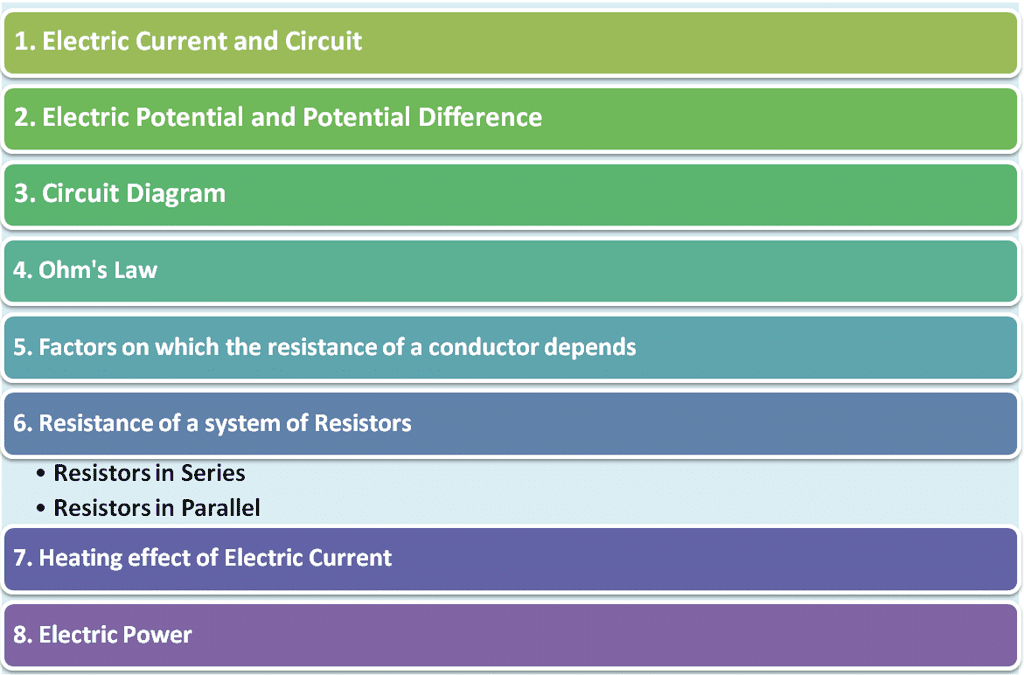Electricity originated from the Greek words “Electrica” and “Elektron”. The greek philosopher Thales was the first to observe the attracting capacity of certain materials when rubbed together.

1. Electric Current and Circuit

• Electricity is one of the most convenient and widely used forms of energy in the world.
• Conductors are those substances in which electric charge can flow freely. Metals are electric conductors. Copper and aluminium are the two most commonly used metals in electricity.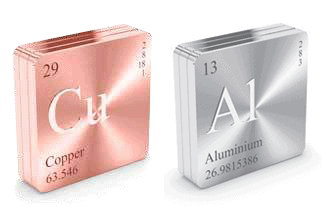• Electrical insulators are those materials in which electric charges do not move freely. Rubber, glass and plastic are examples of insulators.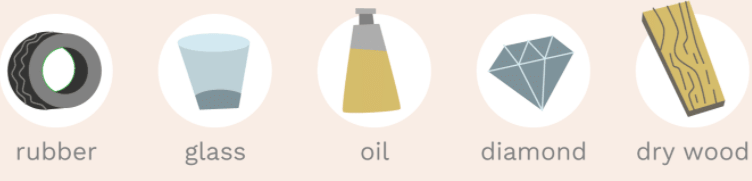Electrical Insulators
• Current electricity is the electricity which deals with moving charges.
• A continuous and closed path of an electric current is called an electric circuit.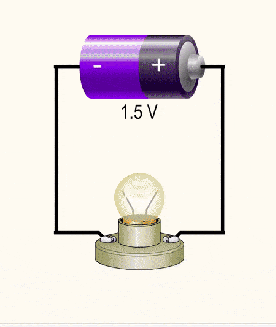Electric Circuit
• Electric current is defined as the rate of flow of electric charge through a cross-section of a conductor.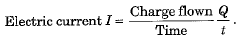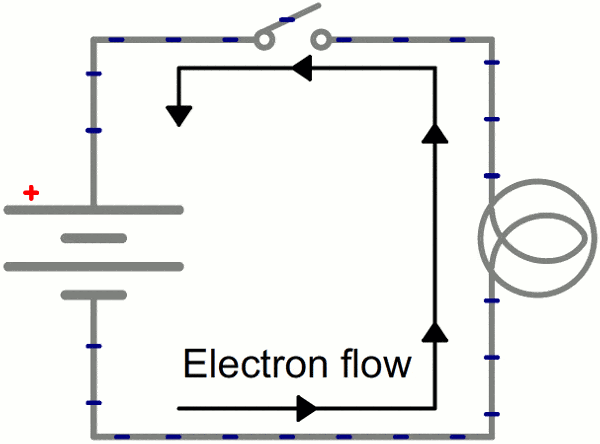The flow of electric current

• S.I. unit of electric current is ‘ampere' (A). One-ampere current is equivalent to the flow of 1 coulomb of charge per second through a section of a conductor. Thus, 1 A = 1 C s-1.
• Direction of flow of electric current is taken as opposite to the direction of the flow of negatively charged electrons. In other terms, the direction of conventional current is taken as the direction along which positive charges will move, if allowed to do so.
• In conductors flow of electric current is due to the flow of electrons. The flow of 6.25 x 1018 electrons per second across a cross-section of a conductor constitutes a current of 1 ampere.

Question for Chapter Notes: Electricity
Try yourself:Which of the given is the SI Unit of Electric Current?

2. Electric Potential & Potential Difference

• The flow of electric charge in a circuit is similar to water flow in a pipe
• The water pipe is analogous to the electric conductor and the amount of water flowing across a section per second corresponds to electric current.
• As water flows from higher pressure (level) to lower pressure (level), the electric current flows from higher electric potential to lower electric potential.
• The potential difference across a conductor is maintained by using a cell or battery.
• The chemical action within a cell generates the potential difference between its electrodes, due to which current flows through the circuit.
• Potential difference between two points in an electric circuit carrying current is defined as the work done to move a unit positive charge from one point to another. If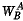be the amount of work done in taking a charge Q from point B to point A, then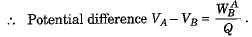• The SI unit of electric potential difference is volt (V), where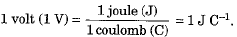3. Circuit Diagram

• A circuit diagram is a simplified representation of the components of an electrical circuit using either the images of the distinct parts or standard symbols.
• It shows the relative positions of all the elements and their connections to one another.
• It is often used to provide a visual representation of the circuit to an electrician. The following figure shows a simple circuit diagram.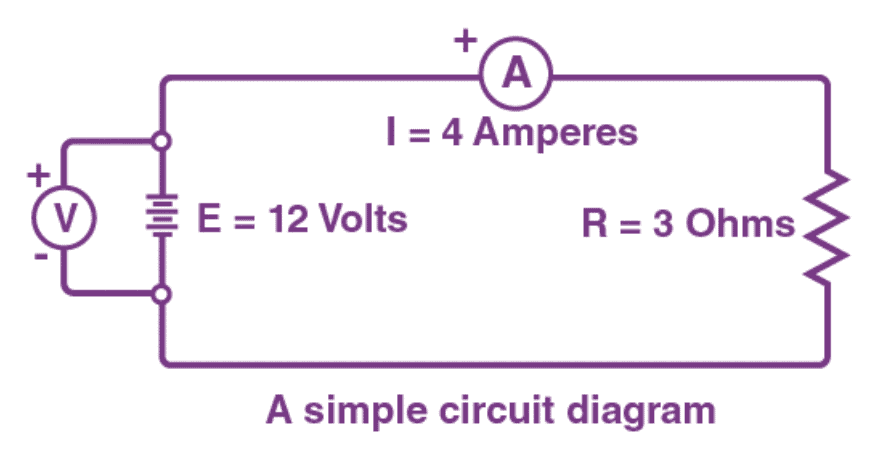Components of Circuit Diagram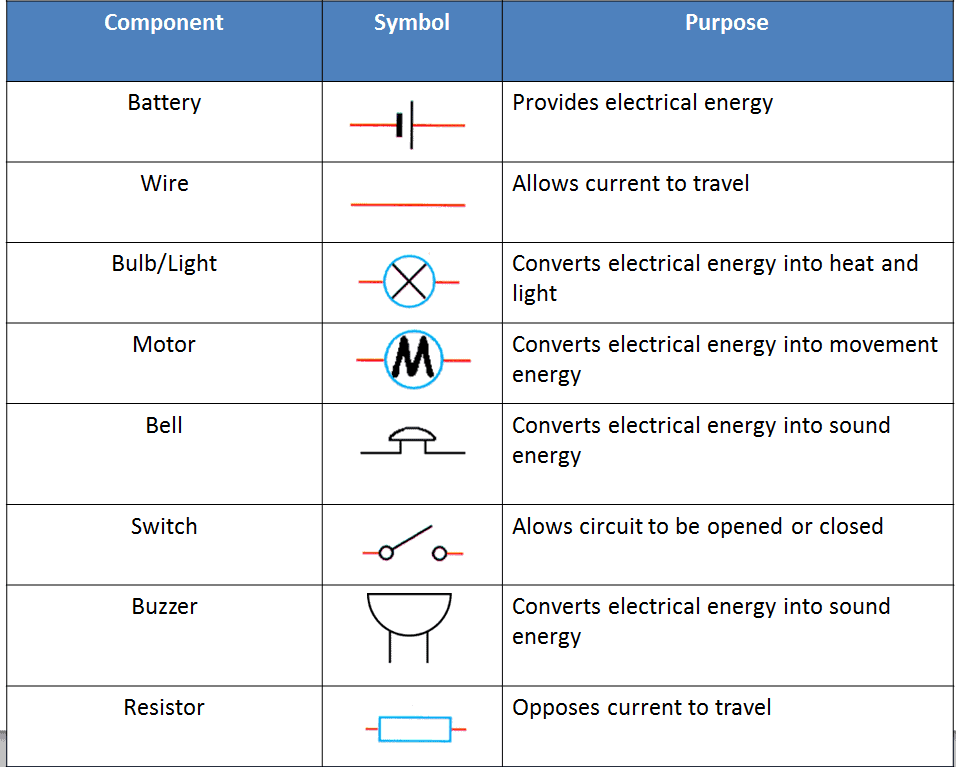4. Ohm's Law

• George Simon Ohm established a relationship between the electric current flowing through a conductor and the potential difference across its ends, due to which current flows.
• According to Ohm’s law, the temperature remaining constant, the current passing through a conductor is directly proportional to the potential difference across its ends, i.e.,

V ∝ I or V = IR

• Here, constant R is known as the resistance of the given conductor. For a given conductor its resistance is constant at a given temperature.
• Electrical resistance of a conductor may be considered as a measure of the opposition offered by it for the flow of electric charge through it.

Mathematically, Resistance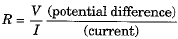• S.I. unit of electrical resistance is oh(Ω), where 1 Ω = 1V/1A.

Question for Chapter Notes: Electricity
Try yourself:The obstruction offered by material of conductor to the passage of electric current is known as:

5. Factors Effecting Resistance of a Conductor

• At a given temperature resistance of a conductor depends on its:
(i) Length L
(ii) Cross-section area A
(iii) Nature of the material of the conductor.
• It is found that R ∝ L and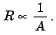Mathematically, R = ρL/A

• Where ρ is a constant known as the resistivity of the material of the conductor, its value depends only on the nature of the material of the conductor and the temperature and is independent of the dimensions (i.e., length and cross-section area) of the conductor.
• The resistivity of a given material is defined as the resistance offered by a cube of that material of side 1 m when current flows perpendicular to the opposite faces. Its unit is ohm-metre (Ω m).
• Metals and alloys have low resistivity in the range of 10-8 Ω m to 10-6 Ω m and are good conductors of electricity.
• Insulators like rubber and glass have a resistivity of the order of 1012 to 1017 Ω m.
• Resistance, as well as the resistivity of a material, vary with temperature. For metallic conductors resistance as well as resistivity increases with increase in temperature.
• Resistivity of pure metals is very-very low. On account of this reason, metals like copper and aluminium are used for electrical transmission lines.
• Resistivity of alloys is greater than that of pure metals. However, alloys do not oxidise easily, and their change in resistivity with rise in temperature is small. Due to these properties, they are used as electrical elements in most of the electric appliances. Generally, nichrome elements are used in electric iron, heater, radiator, geyser etc.
• Tungsten filaments are used in electric lamps because the melting point of tungsten is extremely high.
• A rheostat is a variable resistance device used in electrical circuits. It is used to regulate the current in the circuit without changing the voltage source.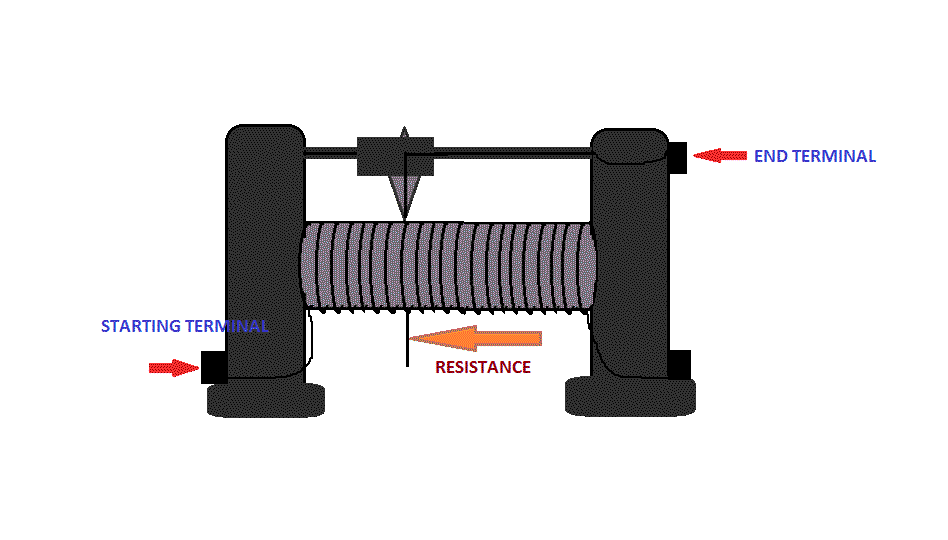A Rheostat
• An ammeter is an instrument which can measure the electric current flowing in an electric circuit directly in amperes and its submultiples.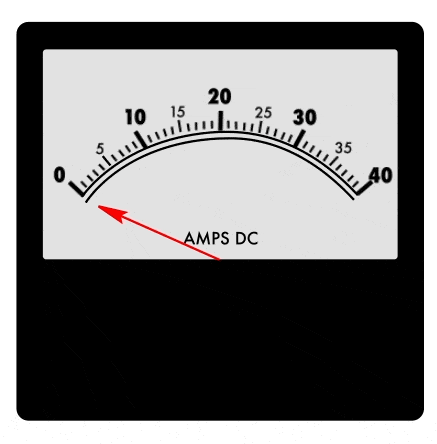Ammeter

• A voltmeter is an instrument used to measure the potential difference across two given points in an electrical circuit directly in volts.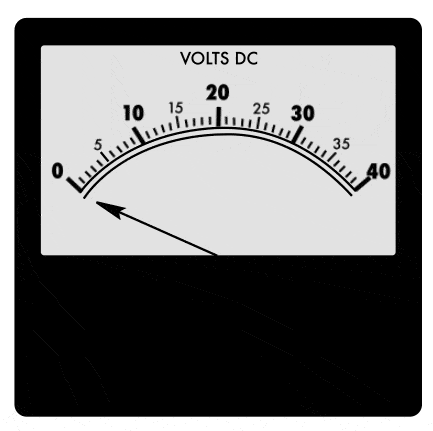Voltmeter

6. Resistance of System of Resistors

• In an electric circuit resistances may be connected in:
(i) Series
(ii) Parallel Arrangement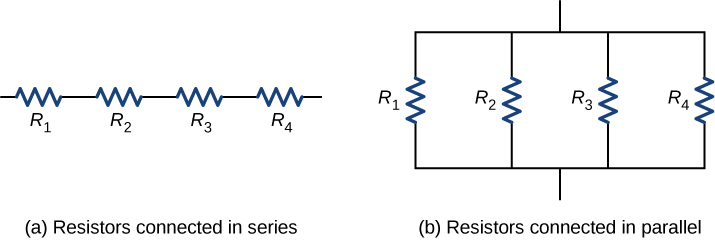• In the series grouping of resistances:
(i) The current flowing through all the resistances is the same.
(ii) The total voltage across the combination is equal to the sum of the voltage drops across the individual resistors.
(iii) The total resistance of the combination is equal to the sum of the individual resistances.
• If R1, R2, R3,... be the individual resistors joined in series then the equivalent resistor Rs is given by Rs = R1 + R2 + R3 + ...
• In the parallel grouping of resistances:
(i) The voltage across each resistor is the same and equal to the voltage across the whole combination.
(ii) The currents in various resistors are inversely proportional to the resistances, and the total current is the sum of the currents flowing through different resistances, and (Hi) the sum of the reciprocals of the separate resistances is equal to the reciprocal of equivalent resistance. If R1, R2, R3,... be the individual resistors joined in parallel then the equivalent resistance Rp is given as :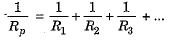• In household electric circuits, a series circuit is not followed. It is because if one component of the series circuit fails, the entire circuit is broken and none of the components works. Moreover, the same current flows through all the components irrespective of their operating needs.
• A parallel circuit divides the current in various components (appliances), and each component can draw current as per its appropriate operation. Separate on/off switch can be put with each component in the parallel circuit. Moreover, the total resistance in parallel circuit arrangement decreases and hence a stronger current can be drawn from a voltage source. Due to these reasons, all appliances are connected in parallel in our household electric circuit.

7. Heating Effect of Electric Current

• If on applying, a potential difference V across the ends of a conductor of resistance R, the current I flows for a time t, then as per Joule’s law of heating the electric energy consumed is given by: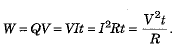• Generally, it is convenient to use the formula W = I2Rt for series-connected circuits because the current I is same in all resistors. For parallel arrangement, we prefer to use the relation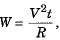because here V is the same across all branches of the circuit.
• The dissipated electrical energy reappears as heat. Thus, the heat produced: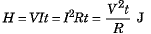Practical Applications of Heating Effect of Electric Current

The heating effect of electric current is used in a large number of devices like electric iron, electric toaster, electric oven, electric kettle, electric heater, electric radiator, geyser etc.

• Electric heating is also used to produce light in electric bulbs. As bulb filament operates at very high temperature, the filament should be thermally insulated. The bulb is filled with chemically inactive nitrogen and argon gases to prolong the life of bulb filament.
• Electric fuse protects an electric circuit or appliance by stopping the flow of any unduly high electric current.
• A fuse consists of a piece of wire of a metal or an alloy of appropriate melting point and is placed in series with the circuit.
• If a current larger than specified value flows through the circuit, the temperature of fuse wire rises beyond its melting point, and fuse melts. As a result, circuit breaks.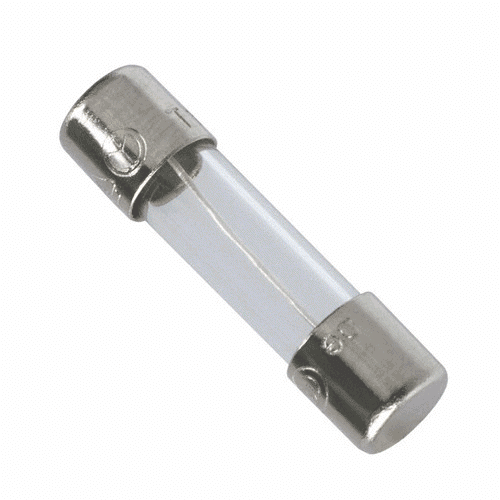• The fuse of appropriate current capacity is used in an electric circuit.

8. Electric Power

• The time rate of doing electric work is called electric power. Thus,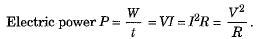• SI unit of electric power is watt (W), where 1 watt = 1-volt * 1 ampere
• A watt-hour and kilowatt-hour are practical units of electric energy. The commercial unit of electric energy is a kilowatt-hour (kW h), where

1 kW h = 1000 Wh = 3.6 x 106 J.

Question for Chapter Notes: Electricity
Try yourself:Amount of energy delivered by a power of one kilowatt in one hour is called _______

Q.1: Why is resistance more in series combination?
Ans: We know R ∝ L. In series combination of resistors, the effective length of the conductor increases, so the resistance increases.

Q.2: Why is it not advisable to handle high voltage electrical circuit with wet hands?
Ans:
The resistance of dry-skin human body is about 50,000 Ω. When the skin is wet, the resistance gets lowered to about 10,000 Ω. If a person with wet hands touches the electrical circuit, high current will flow through the body causing risk of life.

Q.3: How does use of a fuse wire protect electrical appliances?
Ans:
If a current larger than a specified value flows in a circuit, temperature of fuse wire increase its melting point. The fuse wire melts and the circuit breaks.

The document Chapter Notes: Electricity - Notes | Study Science Class 10 - Class 10 is a part of the Class 10 Course Science Class 10.
All you need of Class 10 at this link: Class 10

## Science Class 10

82 videos|348 docs|162 tests
 Use Code STAYHOME200 and get INR 200 additional OFF

## Science Class 10

82 videos|348 docs|162 tests

Track your progress, build streaks, highlight & save important lessons and more!

,

,

,

,

,

,

,

,

,

,

,

,

,

,

,

,

,

,

,

,

,

;